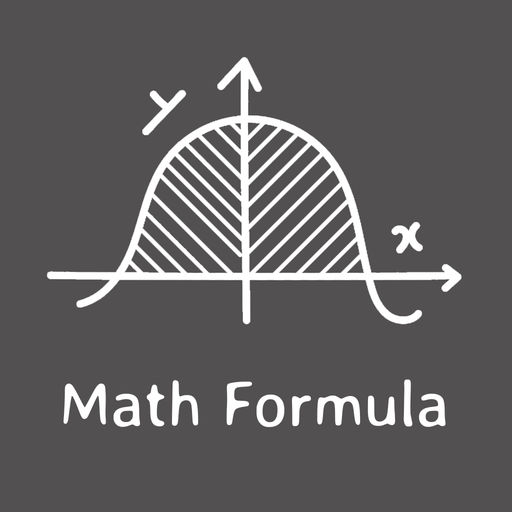Maths Formula# Maths Formula

\$0.99
0

0 Ratings

Update Date

2019-07-19

Size

41.6 MB

### Screenshots for iPhone

iPhone
Maths Formula Description
Want to calculate of area, volume etc of circle, cylinder etc than here is the application for you where you can easily calculate this things by using maths geometry formula.

Application Supported Calculation

• Perimeter of a Square
• Perimeter of a Rectangle
• Area of a Square
• Area of a Rectangle
• Area of a Triangle
• Area of a Trapezoid
• Area of a Circle
• Circumference of a Circle
• Surface Area of a Cube
• Surface Area of a Cylinder
• Volume of a Cylinder
• Surface Area of a Cone
• Volume of a Cone
• Surface Area of a Sphere
• Volume of a Sphere

You have to just enter value of parameter like radius, length, height etc.. and you will get area, volume etc.. of particular objects.
Also in this application you can view last there year of Math's Exam Question paper and Answer Paper of CBSE Class 10 and Class 12.
Maths Formula 1.0 Update
2019-07-19 Version History
More
Price:
\$0.99
Version:
1.0
Size:
41.6 MB
Genre:
Education
Update Date:
2019-07-19
Developer:
Abhayrajsinh Vala
Language:
English
More
You May Also Like
Developer Apps